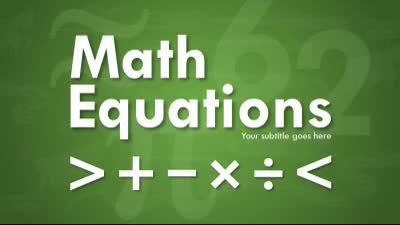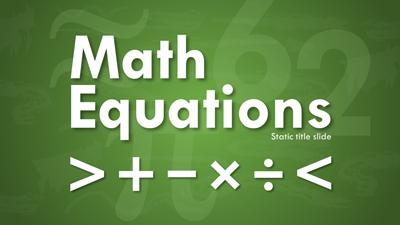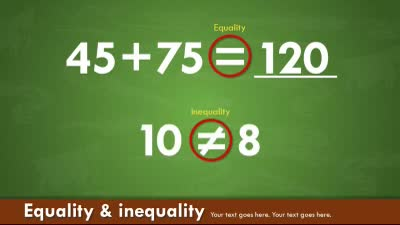X

Math Equations# Math Equations

## Type: PowerPoint Templates

POWERPOINT
2010-2019
PP 365
(PC & MAC)
KEYNOTE
(MAC)
Want this PowerPoint Template?

Get Started Now!

## Description:

This animated widescreen math equations themed template is an excellent way to present simple or complex math equations to your audience or classroom. You can use the provided symbols to create your own equations. Download for PowerPoint or Keynote.

## Keywords

math equations equation add subtract divide multiply pi 3.14 math mathematics geometry algebra square root exponent power equal greater than less than addition subtraction multiplication division equality angle right angle spherical angle measured angle degree arcminute arcsecond ray arc perpendicular parallel similarity equivalence equal by definition approximately equal approximately equal proportional to infinity symbol lemniscate approximation function of x school education teacher teach widescreen hd

Similar Templates and Designs

## Your presentations are going to be amazing!See Plans and Pricing

© 2009-2021 Eclipse Digital Imaging, Inc.

Quality PowerPoint Templates, Animations, videos, and 3D Clipart.
PowerPoint® is a registered trademark of Microsoft Corporation.